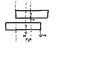# Einstein's Box Change in Centre of Mass

• unscientific
In summary, the conversation is discussing the concept of compensation of shift in centre of mass. The question involves a box of mass M moving leftwards and a small mass m being added to the right end to prevent the centre of mass from moving left. The difference between the attempted solution and the given explanation is the choice of reference point - one being a fixed point in space and the other being the left end of the box. The question raises the question of whether to choose a reference point that is fixed in space or in the same frame of reference. A thought experiment is used to illustrate this concept, but it does not seem to apply in this particular scenario.

## Homework Statement

The question is posted here: http://galileo.phys.virginia.edu/classes/252/mass_and_energy.html

I'm not too convinced by their explanation of 'compensation of shift in centre of mass'

## The Attempt at a Solution

What I understand is this:

1)Box of mass M moves distance d leftwards
2)To prevent centre of mass moving to the left, a small mass m is 'added' to the right end

Then shouldn't it be:

M(d) = (L/2 - d)m

The difference between my answer and theirs is that:

I take the reference point to be an absolute, fixed point in space - the centre of the Box initially.
However, their reference point is the left-end of the box. But that has clearly moved a distance 'd' leftwards.
This raises the important question:

Do we 1)select a reference point that is fixed in space, unchanging with time OR 2) select a reference point in the same frame of reference (left end of the box in this case)

A simple thought experiment:

Imagine a box of length L, with its centre of mass initially at (0,0). Some time later the box moved 10 units right, with its centre of mass now at (10,0). Taking either (0,0) or left end of the box to be the reference point in this case would yield the same result..

However this doesn't seem to be the case in this experiment..

#### Attachments

•einsteinbox.jpg
9.7 KB · Views: 405
Last edited:
any ideas?